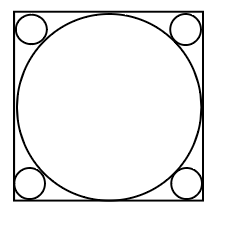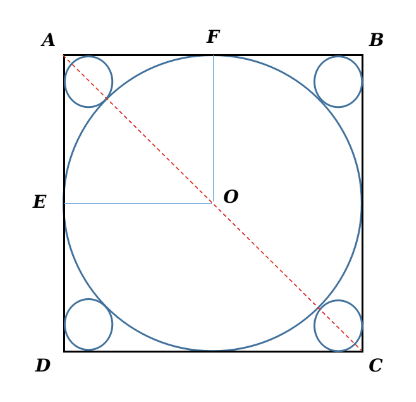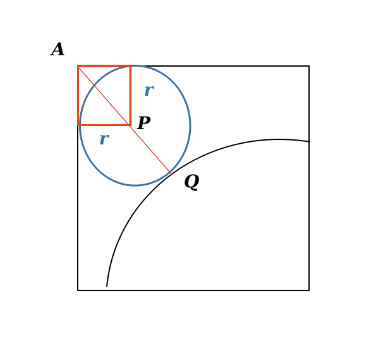Question 8

# In the figure, a circle of radius 2 cm is inscribed in a square. There are four smaller circles at each ofthe cornerof the square. Whatis the total area covered by all the five circles ?SolutionIt has been given that radii OE = OF = 2 cm. Consequently, AB = 4 cm {side of the square}. AC = $$4\sqrt{2}$$ cmFurther, let the radius of the smaller circles be $$r$$ cm. Then, AP = $$r\sqrt{2}$$ cm. Thus, AQ = $$r\sqrt{2}+r$$ or $$r\left(\sqrt{2}+1\right)$$ cm.

There are two such regions on line AC. Hence, we can rewrite AC = $$2\times\ r\left(\sqrt{2}+1\right)+4$$. This is equal to $$4\sqrt{2}$$ cm. On equating, we obtain $$r=\left(6-4\sqrt{2}\right)\$$ cm.

We have been asked to find the total area covered by the five circles = $$4\pi\ +4\times\ \pi\ \left(6-4\sqrt{2}\right)^2\ cm^2$$

Hence, Option A is the correct choice.